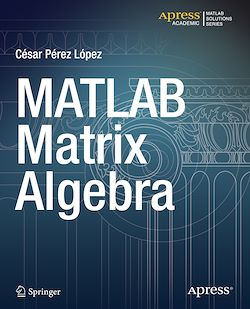# MATLAB Matrix Algebra

-English
240 Pages

Description

MATLAB is a high-level language and environment for numerical computation, visualization, and programming. Using MATLAB, you can analyze data, develop algorithms, and create models and applications. The language, tools, and built-in math functions enable you to explore multiple approaches and reach a solution faster than with spreadsheets or traditional programming languages, such as C/C++ or Java.

MATLAB Matrix Algebra introduces you to the MATLAB language with practical hands-on instructions and results, allowing you to quickly achieve your goals. Starting with a look at symbolic and numeric variables, with an emphasis on vector and matrix variables, you will go on to examine functions and operations that support vectors and matrices as arguments, including those based on analytic parent functions. Computational methods for finding eigenvalues and eigenvectors of matrices are detailed, leading to various matrix decompositions. Applications such as change of bases, the classification of quadratic forms and how to solve systems of linear equations are described, with numerous examples. A section is dedicated to sparse matrices and other types of special matrices. In addition to its treatment of matrices, you will also learn how MATLAB can be used to work with arrays, lists, tables, sequences and sets.

Subjects

##### IT systems

Informations